# Triangle

Triangle. Acute-angled triangle. Right-angled triangle.
Obtuse-angled triangle. Isosceles triangle. Equilateral triangle.
Scalene triangle. Main properties of triangles. Theorems about
congruence of triangles. Remarkable lines and points of a triangle.
Pythagorean theorem. Relation of sides for an arbitrary triangle.

Triangle is a polygon with three sides (or three angles). Sides of triangle are signed often by small letters, corresponding to designations of opposite vertices, signed by capital letters.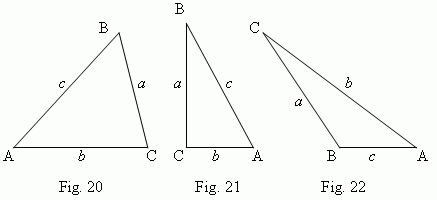If all the three angles are acute ( Fig.20 ), then this triangle is an acute-angled triangle ; if one of the angles is right  (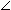C, Fig.21 ), then this triangle is a right-angled triangle ; sides a, b , forming a right angle, are called legs ; side c , opposite to a right angle, called a hypotenuse ; if one of the angles is obtuse  (B, Fig.22 ), then this triangle is an obtuse-angled triangle.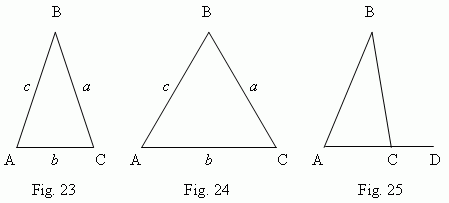A triangle ABC is an isosceles triangle ( Fig.23 ), if the two of its sides are equal ( a = c ); these equal sides are called lateral sides, the third side is called a base of triangle. A triangle ABC is an equilateral triangle ( Fig.24 ), if all of its sides are equal ( a = b = c ). In general case  ( a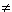bc ) we have a scalene triangle.

Main properties of triangles. In any triangle:

1. An angle, lying opposite the greatest side, is also the greatest angle, and inversely.

2. Angles, lying opposite the equal sides, are also equal, and inversely. In particular,
all angles in an equilateral triangle are also equal.

3. A sum of triangle angles is equal to 180 deg.

From the two last properties it follows, that each angle in an equilateral triangle
is equal to 60 deg.

4.  Continuing one of the triangle sides (AC , Fig. 25), we receive an exterior angleBCD.
An exterior angle of a triangle is equal to a sum of interior angles, not supplementary
with it
:BCD =A  +B.

5. Any side of a triangle is less than a sum of two other sides and more than their
difference
( a < b + c , a > b c ; b < a + c , b > a c ; c < a + b , c > a b ).

Two triangles are congruent, if they have accordingly equal:

a)  two sides and an angle between them;

b)  two angles and a side, adjacent to them;

c)  three sides.

Theorems about congruence of right-angled triangles.

Two right-angled triangles are congruent , if one of the following conditions is valid:

1)  their legs are equal;

2)  a leg and a hypotenuse of one of triangles are equal to a leg and a hypotenuse of another;

3)  a hypotenuse and an acute angle of one of triangles are equal to a hypotenuse and

an acute angle of another;

4)  a leg and an adjacent acute angle of one of triangles are equal to a leg and an

5)  a leg and an opposite acute angle of one of triangles are equal to a leg and an opposite acute angle of another.

Remarkable lines and points of triangle.

Altitude ( height ) of a triangle is a perpendicular, dropped from any vertex to an opposite side ( or to its continuation). This side is called a base of triangle in this case. Three heights of triangle always intersect in one point, called an orthocenter of a triangle . An orthocenter of an acute-angled triangle (point O, Fig.26) is placed inside of the triangle; and an orthocenter of an obtuse-angled triangle (point O, Fig.27) outside of the triangle; an orthocenter of a right-angled triangle coincides with a vertex of the right angle.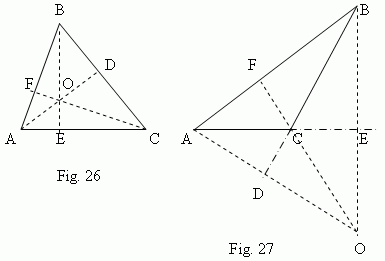Median is a segment, joining any vertex of triangle and a midpoint of the opposite side. Three medians of triangle ( AD, BE, CF, Fig.28 ) intersect in one point O (always lied inside of a triangle), which is a center of gravity of this triangle. This point divides each median by ratio 2:1, considering from a vertex.

Bisector is a segment of the angle bisector, from a vertex to a point of intersection with an opposite side. Three bisectors of a triangle (AD, BE, CF,  Fig.29) intersect in the one point (always lied inside of triangle), which is a center of an inscribed circle (see the section “Inscribed and circumscribed polygons”).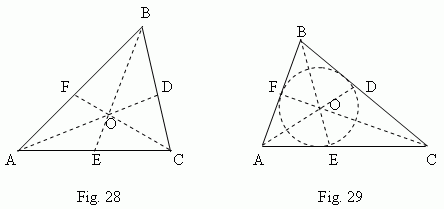A bisector divides an opposite side into two parts, proportional to the adjacent sides ; for instance, on Fig.29 AE : CE = AB : BC .

Midperpendicular is a perpendicular, drawn from a middle point of a segment (side).Three midperpendiculars of a triangle ( ABC,  Fig.30 ), each drawn through the middle of its side ( points K, M, N,  Fig.30 ), intersect in one point O, which is a center of circle, circumscribed around the triangle ( circumcircle ).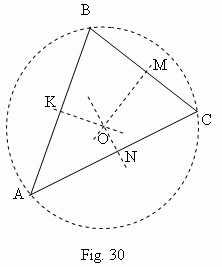In an acute-angled triangle this point lies inside of the triangle; in an obtuse-angled triangle - outside of the triangle; in a right-angled triangle - in the middle of the hypotenuse. An orthocenter, a center of gravity, a center of an inscribed circle and a center of a circumcircle coincide only in an equilateral triangle.

Pythagorean theorem. In a right-angled triangle a square of the hypotenuse length is equal to a sum of squares of legs lengths.

A proof of Pythagorean theorem is clear from  Fig.31. Consider a right-angled triangle ABC with legs a, b and a hypotenuse c.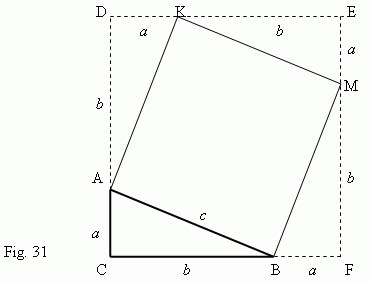Build the square AKMB, using hypotenuse AB as its side. Then continue sides of the right-angled triangle ABC so, to receive the square CDEF, the side length  of which is equal to a + b . Now it is clear, that an area of the square CDEF is equal to ( a + b ) ² . On the other hand, this area is equal to a sum of areas of  four right-angled triangles and a square AKMB, that is

c ² + 4 ( ab / 2 ) = c ² + 2 ab ,

hence,

c ² + 2 ab = ( a + b ) ² ,

and finally, we have:

c ² = a ² + b ² .

Relation of sides’ lengths for arbitrary triangle.

In general case ( for any triangle ) we have:

c ² = a ² + b ² – 2 ab · cos C,

where C – an angle between sides a and b .